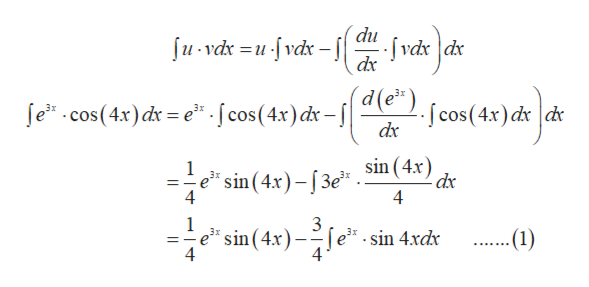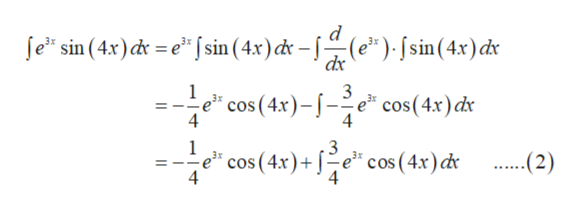# Use the fact that cos(z) = R(eiz) = (eiz + e-iz)/2 to find the integral of (e3xcos(4x))dx.

Question
1 views

Use the fact that cos(z) = R(eiz) = (eiz + e-iz)/2 to find the integral of (e3xcos(4x))dx.

check_circle

Step 1

Here the given integral in not in the complex form so we calculate the integral as it is given in the question:

Step 2

Here we integral the function in the by applying the integration by parts:help_outlineImage Transcriptionclosedu - |fvdx dx dx ſu - vdx =u -[ vdxr d(e*). ſe* .cos(4x) dr = e* [cos(4x)dx - | dx [ cos(4x) dx de sin (4x) dx 4 =e* sin(4x)–[3e" - 4 3 fe* - sin 4xdx =-e" sin (4x) – -(1) %3D 4 fullscreen
Step 3

Again do the integration by parts in ...help_outlineImage Transcriptioncloseſe" sin (4.x) c = e* [ sin (4.x) c – (e")- S sin(4x)cx dx 3 e* cos (4x)-J-e* cos(4x)dx 4 4 e* cos (4.x)+ [-e* cos (4x) c .(2) fullscreen

### Want to see the full answer?

See Solution

#### Want to see this answer and more?

Solutions are written by subject experts who are available 24/7. Questions are typically answered within 1 hour.*

See Solution
*Response times may vary by subject and question.
Tagged in

### Calculus